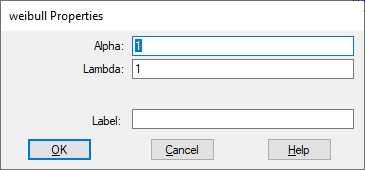## weibull

Block Category: Random Generator

Description: The weibull block generates random numbers from a Weibull distribution. The Weibull distribution is often used in the field of life data analysis due to its flexibility—it can mimic the behavior of other statistical distributions such as the normal and the exponential. If the failure rate decreases over time, then k < 1. If the failure rate is constant over time, then k = 1. If the failure rate increases over time, then k > 1.

An understanding of the failure rate may provide insight as to what is causing the failures:

A decreasing failure rate would suggest "infant mortality". That is, defective items fail early and the failure rate decreases over time as they fall out of the population.

A constant failure rate suggests that items are failing from random events.

An increasing failure rate suggests "wear out" - parts are more likely to fail as time goes on.

When k = 3.4, then the Weibull distribution appears similar to the normal distribution. When k = 1, then the Weibull distribution reduces to the exponential distribution. For more information, see http://en.wikipedia.org/wiki/Weibull_distribution.Alpha: Specifies the shape of the curve. You can enter a value as a C expression.

Lambda: Specifies the scale of the curve. As you increase the value of Lambda, curve is stretched. You can enter a value as a C expression.

Label: Indicates a user-defined block label that appears when View > Block Labels is activated.